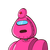# Find the length of diagonal of rectangle having sides 11 cm and 60 cm​

Find the length of diagonal of rectangle having sides 11 cm and 60 cm​

### 1 thought on “Find the length of diagonal of rectangle having sides 11 cm and 60 cm​”

1.We know all angles of a rectangle are 90°

Thus we will use simply Pythagoras Theorem

let the length of the diagonal be x cm

Then

=> x = v11² + 60²

=> x = V121 + 3600

=> x = V3721

=> X = 61cm

Thus the length of the diagonal is 61cm

• ThanKuuuuU ♥️